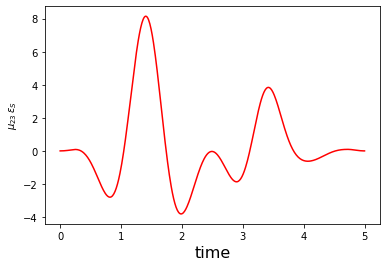# Optimization of a Dissipative State-to-State Transfer in a Lambda System¶

:

# NBVAL_IGNORE_OUTPUT
import os
import qutip
import numpy as np
import scipy
import matplotlib
import matplotlib.pylab as plt
import krotov
import qutip
from qutip import Qobj
import pickle
from functools import partial
%watermark -v --iversions

matplotlib       3.1.2
numpy            1.17.2
krotov           1.0.0
qutip            4.4.1
matplotlib.pylab 1.17.2
scipy            1.3.1
CPython 3.7.3
IPython 7.10.2


$$\newcommand{tr}{\operatorname{tr}} \newcommand{diag}{\operatorname{diag}} \newcommand{abs}{\operatorname{abs}} \newcommand{pop}{\operatorname{pop}} \newcommand{aux}{\text{aux}} \newcommand{opt}{\text{opt}} \newcommand{tgt}{\text{tgt}} \newcommand{init}{\text{init}} \newcommand{lab}{\text{lab}} \newcommand{rwa}{\text{rwa}} \newcommand{bra}{\langle#1\vert} \newcommand{ket}{\vert#1\rangle} \newcommand{Bra}{\left\langle#1\right\vert} \newcommand{Ket}{\left\vert#1\right\rangle} \newcommand{Braket}{\left\langle #1\vphantom{#2}\mid{#2}\vphantom{#1}\right\rangle} \newcommand{ketbra}{\vert#1\rangle\!\langle#2\vert} \newcommand{op}{\hat{#1}} \newcommand{Op}{\hat{#1}} \newcommand{dd}{\,\text{d}} \newcommand{Liouville}{\mathcal{L}} \newcommand{DynMap}{\mathcal{E}} \newcommand{identity}{\mathbf{1}} \newcommand{Norm}{\lVert#1\rVert} \newcommand{Abs}{\left\vert#1\right\vert} \newcommand{avg}{\langle#1\rangle} \newcommand{Avg}{\left\langle#1\right\rangle} \newcommand{AbsSq}{\left\vert#1\right\vert^2} \newcommand{Re}{\operatorname{Re}} \newcommand{Im}{\operatorname{Im}} \newcommand{toP}{\omega_{12}} \newcommand{toS}{\omega_{23}}$$

This example illustrates the use of Krotov’s method with a non-Hermitian Hamiltonian. It considers the same system as the previous example, a transition $$\ket{1} \rightarrow \ket{3}$$ in a three-level system in a $$\Lambda$$-configuration. However, here we add a non-Hermitian decay term to model loss from the intermediary level $$\ket{2}$$. At a technical level, this examples also illustrates how to use args in time-dependent control fields (“QuTiP style”), as opposed to hard-coding parameters or setting them through a closure, as was done in the previous examples.

## The effective Hamiltonian¶

We consider the system as in the following diagram: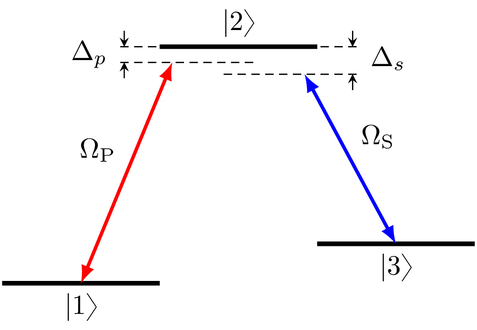with the Hamiltonian

$\begin{split}\Op{H}_{\text{lab}} = \begin{pmatrix} E_1 & -\mu_{12} \epsilon_P(t) & 0 \\ -\mu_{12} \epsilon_P(t) & E_2 & - \mu_{23} \epsilon_S(t) \\ 0 & -\mu_{23} \epsilon_S(t) & E_2 \end{pmatrix}\end{split}$

in the lab frame.

However, we now also include that the level $$\ket{2}$$ decays incoherently. This is the primary motivation of the STIRAP scheme: through destructive interference it can keep the dynamics in a “dark state” where the population is transferred from $$\ket{1}$$ to $$\ket{3}$$ without ever populating the $$\ket{2}$$ state. A rigorous treatment would be to include the dissipation as a Lindblad operator, and to simulate the dynamics and perform the optimization in Liouville space. The Lindblad operator for spontaneous decay from level $$\ket{2}$$ with decay rate $$2\gamma$$ is $$\Op{L} = \sqrt{2\gamma} \ketbra{1}{2}$$. However, this is numerically expensive. For the optimization, it is sufficient to find a way to penalize population in the $$\ket{2}$$ state.

Motivated by the Monte-Carlo Wave Function (MCWF) method, we define the non-Hermitian effective Hamiltonian

$\Op{H}_{\text{eff}} = \Op{H}_{\text{lab}} - \frac{i}{2} \Op{L}^{\dagger} \Op{L}$

In explicit form, this is

$\begin{split}\Op{H}_{\text{eff}} = \begin{pmatrix} E_1 & -\mu_{12} \epsilon_P(t) & 0 \\ -\mu_{12} \epsilon_P(t) & E_2 - i \gamma & - \mu_{23} \epsilon_S(t) \\ 0 & -\mu_{23} \epsilon_S(t) & E_2 \end{pmatrix}\end{split}$

The only change is that the energy of level $$\ket{2}$$ now has an imaginary part $$-\gamma$$, which causes an exponential decay of any population amplitude in $$\ket{2}$$, and thus a decay in the norm of the state. In the MCWF, this decay of the norm is used to track the probability that quantum jump occurs (otherwise, the state is re-normalized). Here, we do not perform quantum jumps or renormalize the state. Instead, we use the decay in the norm to steer the optimization. Using the functional

$J_{T, \text{re}} = 1 - \Re{\Braket{\Psi(T)}{\Psi^{\tgt}}}$

to be minimized, we find that the value of the functional increases if $$\Norm{\ket{\Psi(T)}} < 1$$. Thus, population in $$\ket{2}$$ is penalized, without any significant numerical overhead.

The decay rate $$2\gamma$$ does not necessarily need to correspond to the actual physical lifetime of the $$\ket{2}$$ state: we can choose an artificially high decay rate to put a stronger penalty on the $$\ket{2}$$ level. Or, if the physical decay is so strong that the norm of the state reaches effectively zero, we could decrease $$\gamma$$ to avoid numerical instability. The use of a non-Hermitian Hamiltonian with artificial decay is generally a useful trick to penalize population in a subspace.

The new non-Hermitian decay term remains unchanged when we make the rotating wave approximation. The RWA Hamiltonian now reads

$\begin{split}\Op{H}_{\text{RWA}} = \begin{pmatrix} \Delta_P & -\frac{1}{2} \Omega_P(t) & 0 \\ -\frac{1}{2} \Omega_P^*(t) & -i \gamma & -\frac{1}{2} \Omega_S(t) \\ 0 & -\frac{1}{2} \Omega_S^*(t) & \Delta_S \end{pmatrix}\,,\end{split}$

with complex control fields $$\Omega_P(t)$$ and $$\Omega_S(t)$$, see the previous example. Again, we split these complex pulses into an independent real and imaginary part for the purpose of optimization.

The guess controls are

:

def Omega_P1(t, args):
"""Guess for the real part of the pump pulse.

Blackman shape with amplitude Ω0 from t0P to t0P + ΔTP, with
parameters from args.
"""
t0 = args['t0P']
T = t0 + args['ΔTP']
return args['Ω0'] * krotov.shapes.blackman(t, t_start=t0, t_stop=T)

def Omega_P2(t, args):
"""Guess for the imaginary part of the pump pulse (zero)."""
return 0.0

def Omega_S1(t, args):
"""Guess for the real part of the Stokes pulse.

Blackman shape with amplitude Ω0 from t0S to t0S + ΔTS, with
parameters from args.
"""
t0 = args['t0S']
T = t0 + args['ΔTS']
return args['Ω0'] * krotov.shapes.blackman(t, t_start=t0, t_stop=T)

def Omega_S2(t, args):
"""Guess for the imaginary part of the Stokes pulse (zero)."""
return 0.0


with the Hamiltonian defined as

:

def hamiltonian(args):
"""Lambda-system Hamiltonian in the RWA"""
E1, E2, E3, ΩP, ΩS, γ = (args[key] for key in 'E1 E2 E3 ΩP ΩS γ'.split())

# detunings
ΔP = E1 + ΩP - E2
ΔS = E3 + ΩS - E2

H0 = Qobj([[ΔP, 0.0, 0.0], [0.0, -1j * γ, 0.0], [0.0, 0.0, ΔS]])

HP_re = -0.5 * Qobj([[0.0, 1.0, 0.0], [1.0, 0.0, 0.0], [0.0, 0.0, 0.0]])
HP_im = -0.5 * Qobj([[0.0, 1.0j, 0.0], [-1.0j, 0.0, 0.0], [0.0, 0.0, 0.0]])

HS_re = -0.5 * Qobj([[0.0, 0.0, 0.0], [0.0, 0.0, 1.0], [0.0, 1.0, 0.0]])
HS_im = -0.5 * Qobj([[0.0, 0.0, 0.0], [0.0, 0.0, 1.0j], [0.0, -1.0j, 0.0]])

return [
H0,
[HP_re, Omega_P1],
[HP_im, Omega_P2],
[HS_re, Omega_S1],
[HS_im, Omega_S2],
]


and using the following physical parameters:

:

ARGS = dict(
Ω0=5.0,  # amplitude of both the Pump and the Stokes laser
ΔTP=3.0,  # duration of the Pump laser
ΔTS=3.0,  # duration of the Stokes laser
t0P=2.0,  # starting time for the Pump laser
t0S=0.0,  # starting time for the Stokes laser
t_rise=0.3,  # switch-on/-off time in update shape
E1=0.0,  # energy of level |1⟩
E2=10.0,  # energy of intermediary level |2⟩
E3=5.0,  # energy of target level |3⟩
ΩP=9.5,  # frequency of the Pump laser
ΩS=4.5,  # frequency of the Stokes laser
γ=0.5,  # decay rate on intermediary level |2⟩
T=5.0,  # total process time
)


(cf. the previous example, where these values were hard-coded).

The Hamiltonian is now instantiated as

:

H = hamiltonian(ARGS)


We check the hermiticity of the Hamiltonian:

:

print("H0 is Hermitian: " + str(H.isherm))
print("H1 is Hermitian: "+ str(
H.isherm
and H.isherm
and H.isherm
and H.isherm))

H0 is Hermitian: False
H1 is Hermitian: True


## Define the optimization target¶

We optimize for the phase-sensitive transition $$\ket{1} \rightarrow \ket{3}$$. As we are working in the rotating frame, the target state must be adjusted with an appropriate phase factor:

:

ket1 = qutip.Qobj(np.array([1.0, 0.0, 0.0]))
ket2 = qutip.Qobj(np.array([0.0, 1.0, 0.0]))
ket3 = qutip.Qobj(np.array([0.0, 0.0, 1.0]))

def rwa_target_state(ket3, *, E2, ΩS, T):
return np.exp(1j * (E2 - ΩS) * T) * ket3

psi_target = rwa_target_state(ket3, **{k: ARGS[k] for k in 'E2 ΩS T'.split()})


The objective is now instantiated as

:

objectives = [krotov.Objective(initial_state=ket1, target=psi_target, H=H)]
objectives

:

[Objective[|Ψ₀(3)⟩ to |Ψ₁(3)⟩ via [A₀[3,3], [H₁[3,3], u₁(t)], [H₂[3,3], u₂(t)], [H₃[3,3], u₃(t)], [H₄[3,3], u₄(t)]]]]


## Simulate dynamics under the guess field¶

We use a time grid with 500 steps between $$t=0$$ and $$T=5$$:

:

tlist = np.linspace(0, ARGS['T'], 500)


We propagate once for the population dynamics, and once to obtain the propagated states for each point on the time grid:

:

proj1 = qutip.ket2dm(ket1)
proj2 = qutip.ket2dm(ket2)
proj3 = qutip.ket2dm(ket3)

guess_dynamics = objectives.propagate(
tlist,
propagator=krotov.propagators.expm,
e_ops=[proj1, proj2, proj3],
args=ARGS,
)
guess_states = objectives.propagate(
tlist, propagator=krotov.propagators.expm, args=ARGS,
)

:

def plot_population(result):
fig, ax = plt.subplots()
ax.axhline(y=1.0, color='black', lw=0.5, ls='dashed')
ax.axhline(y=0.0, color='black', lw=0.5, ls='dashed')
ax.plot(result.times, result.expect, label='1')
ax.plot(result.times, result.expect, label='2')
ax.plot(result.times, result.expect, label='3')
ax.legend()
ax.set_xlabel('time')
ax.set_ylabel('population')
plt.show(fig)

def plot_norm(result):

state_norm = lambda i: result.states[i].norm()
states_norm=np.vectorize(state_norm)

fig, ax = plt.subplots()
ax.plot(result.times, states_norm(np.arange(len(result.states))))
ax.set_xlabel('time')
ax.set_ylabel('state norm')
plt.show(fig)

:

plot_population(guess_dynamics)
plot_norm(guess_states)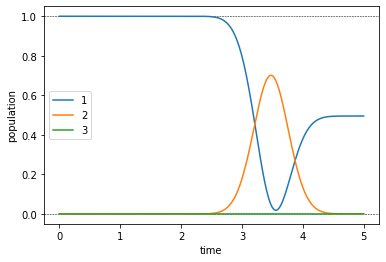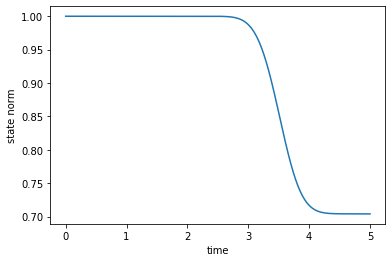The population dynamics and the norm-plot show the effect the non-Hermitian term in the Hamiltonian, resulting in a 30% loss.

## Optimize¶

For each control, we define the update shape and the $$\lambda_a$$ parameter that determines the magnitude of the update:

:

S = partial(  # Scale the *update* of the pulse value at time t
krotov.shapes.flattop,
t_start=0.0,
t_stop=ARGS['T'],
t_rise=ARGS['t_rise'],
func='sinsq',
)

:

pulse_options = {
H: dict(lambda_a=2.0, update_shape=S, args=ARGS),
H: dict(lambda_a=2.0, update_shape=S, args=ARGS),
H: dict(lambda_a=2.0, update_shape=S, args=ARGS),
H: dict(lambda_a=2.0, update_shape=S, args=ARGS)
}


The args here are required to plug in in the parameters in the guess Omega_P1 and Omega_S1. Compare this to the previous example, where the parameters were hardcoded in the definition of the guess controls.

We now run the optimization for 40 iterations, printing out the fidelity

$F_{\text{re}} = \Re{\Braket{\Psi(T)}{\Psi^{\tgt}}}$

after each iteration.

:

def print_fidelity(**kwargs):
F_re = np.average(np.array(kwargs['tau_vals']).real)
print("   F = %f" % F_re)
return F_re

:

opt_result = krotov.optimize_pulses(
objectives, pulse_options, tlist,
propagator=krotov.propagators.expm,
chi_constructor=krotov.functionals.chis_re,
info_hook=print_fidelity,
iter_stop=40
)

   F = -0.007812
F = 0.055166
F = 0.117604
F = 0.178902
F = 0.238507
F = 0.295926
F = 0.350749
F = 0.402648
F = 0.451388
F = 0.496822
F = 0.538882
F = 0.577573
F = 0.612961
F = 0.645161
F = 0.674324
F = 0.700629
F = 0.724268
F = 0.745445
F = 0.764364
F = 0.781226
F = 0.796224
F = 0.809541
F = 0.821349
F = 0.831809
F = 0.841064
F = 0.849250
F = 0.856486
F = 0.862881
F = 0.868532
F = 0.873527
F = 0.877942
F = 0.881847
F = 0.885302
F = 0.888362
F = 0.891074
F = 0.893481
F = 0.895618
F = 0.897519
F = 0.899211
F = 0.900721
F = 0.902071


We look at the optimized controls and the population dynamics they induce:

:

def plot_pulse_amplitude_and_phase(pulse_real, pulse_imaginary,tlist):
ax1 = plt.subplot(211)
ax2 = plt.subplot(212)
amplitudes = [np.sqrt(x*x + y*y) for x,y in zip(pulse_real,pulse_imaginary)]
phases = [np.arctan2(y,x)/np.pi for x,y in zip(pulse_real,pulse_imaginary)]
ax1.plot(tlist,amplitudes)
ax1.set_xlabel('time')
ax1.set_ylabel('pulse amplitude')
ax2.plot(tlist,phases)
ax2.set_xlabel('time')
ax2.set_ylabel('pulse phase (π)')
plt.show()

print("pump pulse amplitude and phase:")
plot_pulse_amplitude_and_phase(
opt_result.optimized_controls, opt_result.optimized_controls, tlist)
print("Stokes pulse amplitude and phase:")
plot_pulse_amplitude_and_phase(
opt_result.optimized_controls, opt_result.optimized_controls, tlist)

pump pulse amplitude and phase: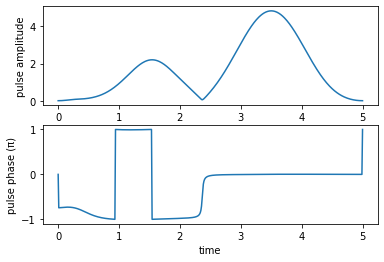Stokes pulse amplitude and phase: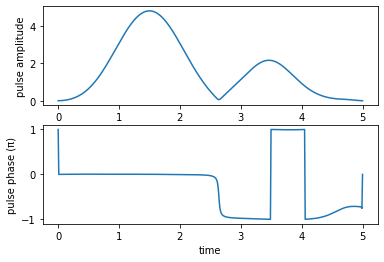We check the evolution of the population due to our optimized pulses.

:

opt_dynamics = opt_result.optimized_objectives.propagate(
tlist, propagator=krotov.propagators.expm, e_ops=[proj1, proj2, proj3])
opt_states = opt_result.optimized_objectives.propagate(
tlist, propagator=krotov.propagators.expm)

:

plot_population(opt_dynamics)
plot_norm(opt_states)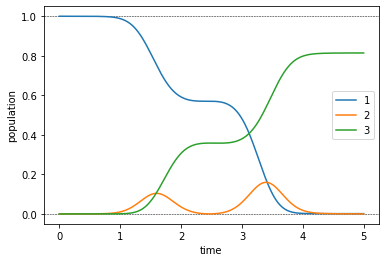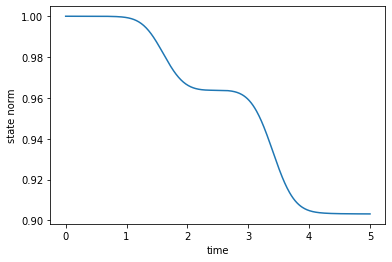These dynamics show that the non-Hermitian Hamiltonian has the desired effect: The population is steered out of the decaying $$\ket{2}$$ state, with the resulting loss in norm down to 10% from the 30% loss of the guess pulses. Indeed, these 10% are exactly the value of the error $$1 - F_{\text{re}}$$, indicating that avoiding population in the $$\ket{2}$$ part is the difficult part of the optimization. Convergence towards this goal is slow, so we continue the optimization up to iteration 2000.

:

dumpfile = "./non_herm_opt_result.dump"
if os.path.isfile(dumpfile):
else:
opt_result = krotov.optimize_pulses(
objectives, pulse_options, tlist,
propagator=krotov.propagators.expm,
chi_constructor=krotov.functionals.chis_re,
info_hook=krotov.info_hooks.chain(print_fidelity),
iter_stop=2000,
continue_from=opt_result
)
opt_result.dump(dumpfile)

:

print("Final fidelity: %.3f" % opt_result.info_vals[-1])

Final fidelity: 0.966

:

def plot_convergence(result):
fig, ax = plt.subplots()
ax.semilogy(result.iters, 1-np.array(result.info_vals))
ax.set_xlabel('OCT iteration')
ax.set_ylabel('error')
plt.show(fig)


To get a feel for the convergence, we can plot the optimization error over the iteration number:

:

plot_convergence(opt_result)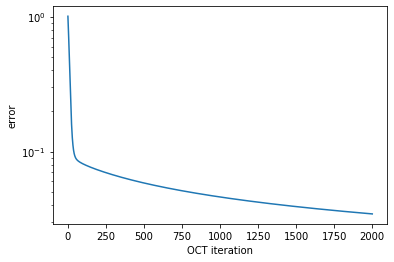We have used here that the return value of the routine print_fidelity that was passed to the optimize_pulses routine as an info_hook is automatically accumulated in result.info_vals.

We also look at optimized controls and the dynamics they induce:

:

print("pump pulse amplitude and phase:")
plot_pulse_amplitude_and_phase(
opt_result.optimized_controls, opt_result.optimized_controls, tlist)
print("Stokes pulse amplitude and phase:")
plot_pulse_amplitude_and_phase(
opt_result.optimized_controls, opt_result.optimized_controls, tlist)

pump pulse amplitude and phase: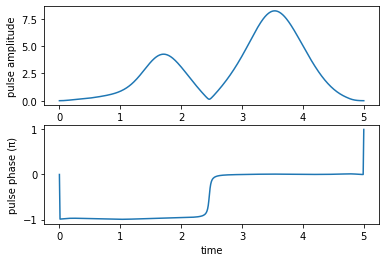Stokes pulse amplitude and phase: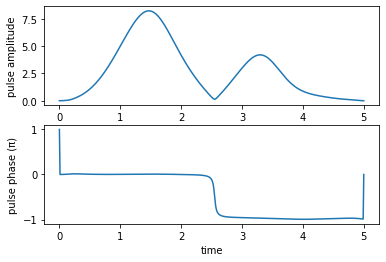:

opt_dynamics = opt_result.optimized_objectives.propagate(
tlist, propagator=krotov.propagators.expm, e_ops=[proj1, proj2, proj3])
opt_states = opt_result.optimized_objectives.propagate(
tlist, propagator=krotov.propagators.expm)

:

plot_population(opt_dynamics)
plot_norm(opt_states)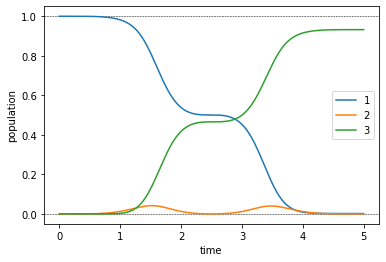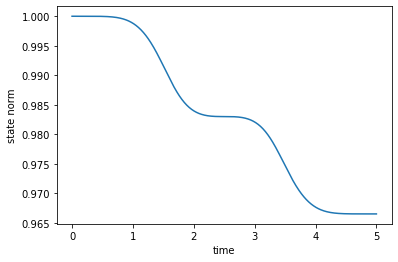In accordance with the lower optimization error, the population dynamics now show a reasonably efficient transfer, and a significantly reduced population in state $$\ket{2}$$.

Finally, we can convert the complex-valued $$\Omega_P$$ and $$\Omega_S$$ functions to the physical electric fields $$\epsilon_{P}$$ and $$\epsilon_{S}$$:

:

def plot_physical_field(pulse_re, pulse_im, tlist, case=None):

if case == 'pump':
w = 9.5
elif case == 'stokes':
w = 4.5
else:
print('Error: selected case is not a valid option')
return

ax = plt.subplot(111)
ax.plot(tlist,pulse_re*np.cos(w*tlist)-pulse_im*np.sin(w*tlist), 'r')
ax.set_xlabel('time', fontsize = 16)
if case == 'pump':
ax.set_ylabel(r'$\mu_{12}\,\epsilon_{P}$')
elif case == 'stokes':
ax.set_ylabel(r'$\mu_{23}\,\epsilon_{S}$')
plt.show()

print('Physical electric pump pulse in the lab frame:')
plot_physical_field(
opt_result.optimized_controls, opt_result.optimized_controls, tlist, case = 'pump')

print('Physical electric Stokes pulse in the lab frame:')
plot_physical_field(
opt_result.optimized_controls, opt_result.optimized_controls, tlist, case = 'stokes')

Physical electric pump pulse in the lab frame: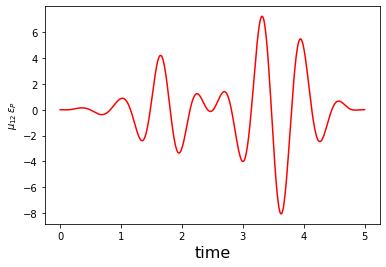Physical electric Stokes pulse in the lab frame: